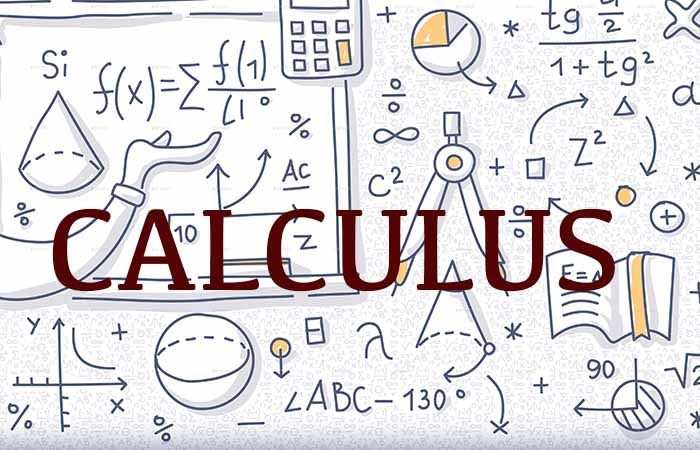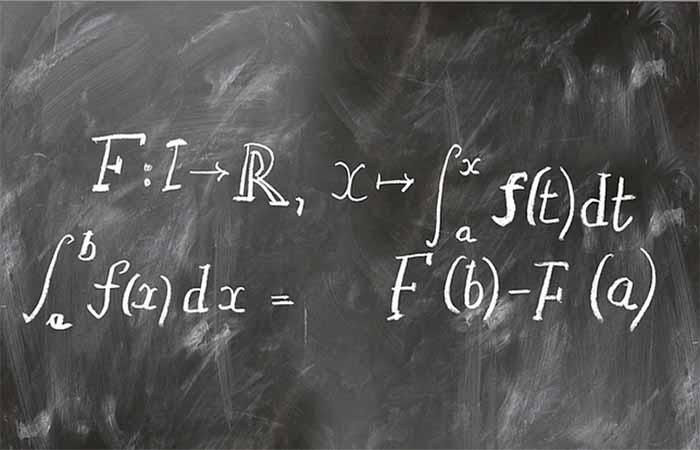# Mathematics

Home/Mathematics## Calculus I

2020-01-23T04:21:14+00:00

Introduction to the primary concepts and techniques of differential and integral calculus. Topics include limits and continuity, the derivative, differentiation and integration of algebraic and trigonometric functions, linearization of functions, Mean Value theorem, extrema and curve sketching, area and the definite integral and fundamental theorem.## Calculus 3

2020-01-22T14:55:32+00:00

Topics include vectors in 2D and 3D-space, curves in space, directional derivatives, gradient, extrema of multivariable functions, Lagrange multipliers, double and triple integrals, integration in cylindrical and spherical coordinates. Emphasis on conceptual understanding and modeling of physical phenomena.